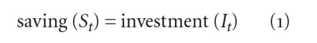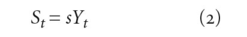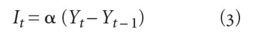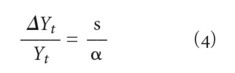# Harrod economic-growth model

## Harrod economic-growth model

a theoretical construct that examines the growth path of the economy Specifically, the model is concerned with the rate at which NATIONAL INCOME (Y) must grow in order to satisfy the Keynesian EQUILIBRIUM NATIONAL INCOME condition:where t denotes a time period.(2) In the model, St depends on national income: i.e. saving in each period depends on the income of the same period; s represents the AVERAGE PROPENSITY TO SAVE and MARGINAL PROPENSITY TO SAVE.

It depends on the rate of change of income from one period to the next, notationally Yt (income in current time period), Yt - 1 (income in previous time period), i.e:i.e. investment is INDUCED INVESTMENT, with the symbol α representing the ACCELERATOR.

Given this assumed saving and investment behaviour, the equilibrium condition represented in equation (1) can be shown to require that:The left-hand expression is the percentage change in income; the right-hand side is the ratio of the marginal propensity to save to the accelerator. Since this is deducted from the condition for maintaining equilibrium in each time period, Harrod called the rate of change in income the ‘warranted’ rate of growth.

If the ‘actual’ rate of growth exceeds (or is lower than) the warranted rate, income will be raised more (or lowered more) than is appropriate for maintaining equilibrium, causing a cumulative deviation from the equilibrium path.

Equation (4) determines the warranted rate of growth. The actual rate of growth is determined in the model by the rate of growth of the LABOUR FORCE and the rate of growth of labour PRODUCTIVITY. Suppose that the labour force is growing at the rate of 1% per year and labour productivity at the rate of 2% per year, the actual (or the ‘natural’ rate of growth, as Harrod called it) attainable rate of growth of national income and output is thus 3% per year.

If the natural rate of growth exceeds the warranted rate of growth, then the economy will experience a cumulative deviation from the warranted rate, income growing at an excessive rate and causing secular expansion. By contrast, if the natural rate falls below that of the warranted rate, then there will be SECULAR STAGNATION. See DOMAR ECONOMIC-GROWTH MODEL, SOLOW ECONOMIC-GROWTH MODEL.

Collins Dictionary of Economics, 4th ed. © C. Pass, B. Lowes, L. Davies 2005
Site: Follow: Share:
Open / Close